# Problem 52809. Easy Sequences 28: Sum of Radicals of Integers

The radical of a positive integer x is defined as the product of the distinct prime numbers dividing x. For example, the distinct prime factors of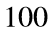is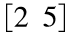, therefore the radical ofis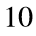. Similarly, the radicals of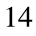,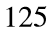and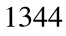are, 5 and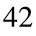, respectively, The number1is considered to be the radical of itself.
Given a limit n, find the sum of the radicals of all positive integers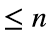.
For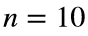, the radicals are: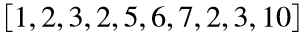. Therefore, the output should be '41'.

### Solution Stats

64.71% Correct | 35.29% Incorrect
Last Solution submitted on Apr 18, 2023

### Community Treasure Hunt

Find the treasures in MATLAB Central and discover how the community can help you!

Start Hunting!